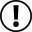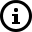# Examples that you can use on the custom formula feature when exporting data out of AZInsight

Below are some examples of formulas or functions that you can use

 What to use =SUM(range) What it does it will add the values of a cell to another cell Example =SUM(A:A+B:B+C:C) How it can be used You can use the sum to add for example two custom costs in columns A and B with the selling price in column C.

 What to use =CONCATENATE(Value1, “ ”,Value2) What it does allows you to combine values of multiple cells into one cell, e.g., first and last name Example =CONCATENATE(A:A&","&B:B) How it can be used You can use the concatenate to join the brand name with a comma followed by the manufacturer name.

 What to use =IF (logical_test, [value_if_true], [value_if_false]) What it does allows you to run a logical test and returns one value for a TRUE result, and another for a FALSE result. Example =IF(B:B<10000,"Good Buy","Not Good Buy") How it can be used You can use the IF statement to compare a rank in a cell to a number you set as the ideal rank of the ASIN you wish to sell and return a Good or Not Good Buy.

 What to use =MIN(value1, [value2, ...]) What it does allows you to find the smallest number in a given range Example =MIN(A:A,B:B) How it can be used You can use the MIN function to find which price is lower between the Lowest FBA Price and the Buybox Price.

 What to use =IMAGE("https://graph.keepa.com/pricehistory.png?asin=ASIN&domain=X&new=X&range=X&salesrank=X&amazon=X") What it does this will display a static sales rank Keepa image graph for a certain ASIN Example =IMAGE("https://graph.keepa.com/pricehistory.png?asin=B09HSPT8ZM&domain=com&new=0&range=365&salesrank=1&amazon=0") How it can be used It is used to display a 365 Sales Rank static Keepa graph.In the examples above we show referencing cells using relative position. You can also use absolute cell references. Referencing cells in Google Spreadsheets and Excel can get complicated. Check out these external resources to learn more about cell referencing:Glossary
- A formula is an expression that calculates values in a cell or a range of cells. For example, =A2+A2+A3+A4.
- A function is a predefined formula already available in Excel. Functions perform specific calculations in a particular order based on the specified values, called arguments, or parameters. For example, instead of specifying each value to be summed like in the above formula, you can use the SUM function to add up a range of cells: =SUM(A2:A4)